## Wednesday, August 22, 2012

### Minkowski Collisions - I Want My Game To Have Slopes!

I'm going to outline how I did the collision detection in my game. I'm not going to provide actual code, just the idea behind each step. You have to code it yourself and work out the issues yourself. You can see a video of this system in action in my previous post.

First I'd like to recommend this brilliant article by David Rosen about using vectors in games. There's lots of interesting stuff there that can help you out and since I'm going to talk about vectors a tiny bit in this guide you can go and get the relevant info there.

### Taking the Minkowksi Difference:

The idea behind my collision detection system is the Minkowski difference. If you're a boss at understanding things (unlike me) you can check out this guide to Minkowski collision detection and ignore the rest of this post. I mostly used it for the flash app on that page to provide some intuition of what Minkowski difference actually does.

Why use Minkowski? What is it?

The Minkowski difference takes two convex polygons and produces a third. The way it does this is by taking the coordinates of each vertex of the first polygon and finding the difference between that and the coordinates of each vertex of the other polygon. This produces a scattered array of points in space.

e.g. The Minkowski difference of {(1,1);(3,1);(2,2)} and {(2,1);(4,1);(3,2)} is:
(1,1) - (2,1) = (-1,0)
(1,1) - (4,1) = (-3,0)
(1,1) - (3,2) = (-2,-1)
(3,1) - (2,1) = (1,0)
(3,1) - (4,1) = (-3,0)
etc...
(2,2) - (3,2) = (-1,0)
You'll end up with 3*3 = 9 points.

We can create a shape using the outermost of these points. If that shape contains the origin, then we know we have a collision. This is our goal. Using polygons for the player and every other object means we can use the Minkowski difference polygons to work out how collisions with these objects would occur.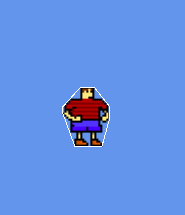The polygon I currently use for Centure

### Bounding the points:

Remove duplicates and finding a start point:
So, as we know, the Minkowski Difference provides this array of points and something you'll notice if you play around with the flash app in the guide I posted above is that several of these points fall exactly over other points. You'll need to remove all of these duplicate points. At the same time it would be a good idea to get the value of the furthest left (or right) of the highest points. You can do both of these in one loop.

We're going to use this top-left/top-right point as the starting point for this algorithm. I put preference on it being highest over it being furthest left. So it will find the highest point, and if there's more than one of this height it will take the one furthest left. This is just preference, all that really matters is that you use any point that you know will be on the outside of the shape and at a vertex.The points from earlier make 9 dots but some overlap so it only looks like 7

Points on a line and lines dividing points:
The idea of this algorithm is to see if a line between two points splits the other points into two groups. This is a pretty simple way to tell if the points lie on the outer edge of the shape we're trying to make.

So we start with our top left point which we know will be a vertex in the shape and we make a line to some other point and see if this line subdivides the remaining points (at least one of those remaining points must be found on each side).Subdivides

Once we find a line that does not do so, we can add this point to our new shape. This is our first edge.Does not subdivide - an edge!

We can continue to do this working from the next point we found and work our way around the polygon. You need to remember to check, after you have three points in the new shape, if you cannot make a line back to the original top-left point that does not subdivide the remaining points. If this occurs you have made your way back to the start and have defined your polygon as a set of points in order from top left all the way around.

Optional code?: I'm not entirely sure, but I added some code to remove co-linear points from the new polygon whilst creating it. I don't think these will affect anything other than having to keep track of more than the minimum amount of points needed to describe the polygon you have created. In theory it should work without doing this but I'd recommend doing it anyway since I know it works.

The last thing you want to do when creating your Minkowski polygon is find a point that you know will always be inside it. I just took the average of all the points. As it is a convex polygon this point will always be inside the polygon.

### Is this a collision?

Line in a shape:
From the point we found inside the Minkowski polygon we create a line segment to the origin. If this line segment crosses any of the sides of the polygon then we know that the origin is not contained in the Minkowski polygon and that the two polygons the Minkowski polygon is created from are not colliding.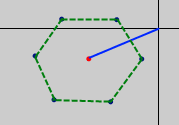This is not a collision

If this line segment is contained within the shape however, we have a collision and we should keep this polygon for later use. Any Minkowski polygons that are not the product of a collision can be destroyed and since it's pretty costly to make them you'd want to only take the Minkowski difference when simpler methods of finding out if objects are near each other can't be sure if there's a collision or not.The line from our point (in red) to the origin does not hit an edge. A collision.

### The direction vector

The final piece of this convoluted puzzle is to work out what to do with the polygon we are using to represent the player to make it exit the polygon it has collided with in a realistic fashion. Here the Minkowski difference polygon becomes extremely useful. This is also the part when you need to think using vectors.

In order to get into the collision your player will need to have had some velocity vector (an arrow the length of how far the player moves each frame).

If you take the velocity vector from the origin and find out which edge of the Minkowski polygon it intercepts you can easily make the perfect exit vector.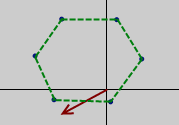Let's say this was the velocity vector in red.

If we take the velocity vector from the origin to the edge it intercepts with...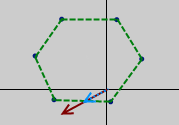We take the blue vector
... and then find the vector projection of that vector onto the edge of the Minkowski polygon it intercepts withHere is the vector projection in grey.
And then if we take the vector projection onto the edge minus the origin-to-edge vector we get the perfect exit vector for the particular collision. You then use this vector to move the player out of the collision in the same frame that he gets into it so it looks like he was never there and that the object he collided with is completely solid.grey vector - blue vector = green exit vector
Feel free to use any of the ideas in this post for your own collision detection system. This is certainly not the only way to do this and I'm sure it's not even a patch on the most efficient. I've tried my best to make this easy to understand. Use the sources at the top if you need more information. Now it's back to work for me. Happy coding!

1.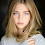2.